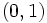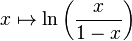# Inverse logistic function

(diff) ← Older revision | Latest revision (diff) | Newer revision → (diff)
The inverse logistic function or log-odds function is a function from the open interval$(0,1)$ to all of$\R$ defined as follows:$x \mapsto \ln \left(\frac{x}{1 - x}\right)$